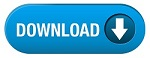Home » SSC » Model Questions for Scientific Assistant (IMD) Exam (Paper-2) PHYSICS – Set 30

# Model Questions for Scientific Assistant (IMD) Exam (Paper-2) PHYSICS – Set 30

## 1. An electromagnetic wave has a wavelength of 1 m. What is its frequency?

a. 30,000 Hz
b. 300,000 Hz
c. 3,000,000 Hz
d. 300,000,000 Hz

## 2. If Proxima Centauri, our closest star, were to explode right now, it would take about 4 years for us to know about it because—-

a. it takes 4 years to build a big telescope to see the star
b. it takes 4 years to obtain funding to build the necessary telescope
c. the star is 4 years old
d. it takes 4 years for the light from this star to reach us\

## 3. When two waves are superposed, they reinforce each other, or cancel each other.

a. Huygen’s Principle
b. Diffraction
c. Interference
d. Polarization

## 4. A two-dimensional interference pattern that captures three-dimensional information.

a. Huygen’s Principle
b. Diffraction
c. Interference
d. Hologram

## 5. Alignment of the transverse vibrations of electromagnetic radiation.

a. Huygen’s Principle
b. Diffraction
c. Interference
d. Polarization

### 6. Every point on a wave front acts as a source of waves. These waves combine to form the next wave front.

a. Huygen’s Principle
b. Diffraction
c. Interference
d. Polarization

## 7. Bending of light around the edges of an object or around the edges of a narrow slit.

a. Huygen’s Principle
b. Diffraction
c. Interference
d. Polarization

## 8. To most efficiently absorb an electromagnetic wave, the frequency of the wave should be ___ the natural frequency of the material being used to absorb the wave.

a. greater than
b. less than
c. equal to
d. zero compared to

## 9. When electron clouds in a material are made to oscillate ____ waves are necessarily emitted—-

a. electronic
b. magnetic
c. de Broglie
d. electromagnetic

a. zero
b. infinite
c. smaller
d. larger

#